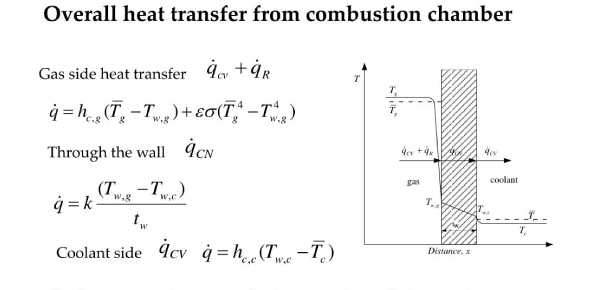# Trivia On Heat Transfer And Combustion! Quiz

20 Questions | Attempts: 484
ShareSettings.

• 1.
Solid X is placed in contact with solid Y.  Heat energy will flow spontaneously from X to Y when
• A.

X is 20°C and Y is 20°C

• B.

X is 10°C and Y is 5°C

• C.

X is -25°C and Y is -10°C

• D.

X is 25°C and Y is 30°C

• 2.
As water warms from 50°C to 85°C, the kinetic energy of the molecules will
• A.

Increase

• B.

Decrease

• C.

Stay the same

• 3.
The temperature of a sample of pudding is a measure of the ___________ of the particles that make up the pudding.
• A.

Activation energy

• B.

Kinetic energy

• C.

Potential energy

• D.

Ionization energy

• 4.
Boiling spaghetti noodles is an example of
• A.

Conduction

• B.

Convection

• C.

• D.

Combustion

• 5.
Using a solar cooker is an example of
• A.

Conduction

• B.

Convection

• C.

• D.

Combustion

• 6.
An egg frying in a skillet is an example of
• A.

Conduction

• B.

Convection

• C.

• D.

Combustion

• 7.
Products of a complete combustion are
• A.

Water and oxygen.

• B.

Water and carbon dioxide.

• C.

Oxygen and carbon dioxide.

• D.

Hydrogen and carbon dioxide.

• 8.
As matter changes from solid to a liquid energy is
• A.

Absorbed

• B.

Released

• C.

Created

• D.

Destroyed

• 9.
What are 2 requirements for a combustion reaction to occur?
• A.

Fuel & oxygen

• B.

Fuel & hydrogens

• C.

Carbon dioxide & oxygen

• D.

Water & oxygen

• 10.
Heat is defined as
• A.

The amount of kinetic energy.

• B.

The amount of potential energy.

• C.

The amount of hotness.

• D.

The amount of coldness.

• 11.
Ice can heat an object
• A.

Never

• B.

If the object is warmer than the ice.

• C.

If the object is colder than the ice.

• D.

Always

• 12.
Heat transfers from ________ to ________
• A.

Hot, cold

• B.

Cold, hot

• C.

Air, water

• D.

Water, air

• 13.
If I stick a metal skewer (stick) into a potato, the amount of cooking time required  will
• A.

Decrease

• B.

Stay the same

• C.

Increase

• 14.
If object X has a temperature of 90° C and object Y has a temperature of 20°C, when they are placed in contact with each other the final temperature of each will be approximately
• A.

90°C

• B.

20°C

• C.

55°C

• D.

110°C

• 15.
As matter changes from a liquid to a solid entropy
• A.

Increases

• B.

Decreases

• C.

Is created

• D.

Is destroyed

• 16.
When a liquid evaporates what cools?
• A.

The liquid

• B.

The gas

• C.

The surroundings

• D.

Nothing

• 17.
A campfire eventually burns out because
• A.

You stop giving it fuel (wood, paper, lighter fluid, etc)

• B.

The air temperature is too low.

• C.

It does not have enough space.

• D.

It gets too hot.

• 18.
As the amount of cooking time increases, the amount of heat transfer
• A.

Stays the same

• B.

Increases

• C.

Decreases

• 19.
Matter  melting _________________ energy.
• A.

Absorbs

• B.

Releases

• C.

Excites

• D.

Destroys

• 20.
Matter condensing is an _______________ process.
• A.

Absorbs

• B.

Releases

• C.

Excites

• D.

Destroys

## Related TopicsBack to top
×

Wait!
Here's an interesting quiz for you.